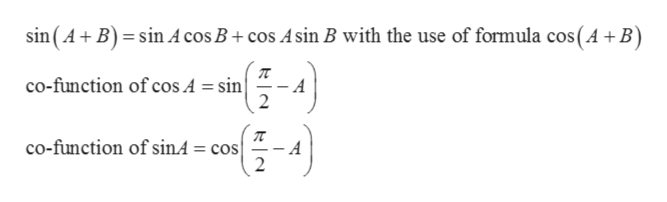# (1) Prove sin(A+B) = sin(A) cos(B)+sin(B) cos(A) by using the formula for cos(A+B)and a cofunction identity.

Question

(1) Prove sin(A+B) = sin(A) cos(B)+sin(B) cos(A) by using the formula for cos(A+B)
and a cofunction identity.

check_circleExpert Solution
Step 1

Given:

Step 2

We are going to p...help_outlineImage Transcriptionclosesin (A B) sin A cos B+ cos Asin B with the use of formula cos (A +B) co-function of cos A = sin A 2 co-function of sinA = cos - A 2 fullscreen

### Want to see the full answer?

See Solution

#### Want to see this answer and more?

Solutions are written by subject experts who are available 24/7. Questions are typically answered within 1 hour*

See Solution
*Response times may vary by subject and question
Tagged in

### Other